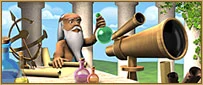ExperimentsScience research
Research Points:
Requires:
Accumulated: ()
Requires:
 : Optics (Research)
Allows:
 : Mechanical Pen (Research) Swapping Crystal Glass for research points Technocracy (Government)
Scores:
Scientists (Accumulated)
427 (1,561)
Levels of research:
4

## Description

Our scientists want to have the chance of testing their theories in practice.  These trials would most probably accelerate research time, but does mean they would need a certain amount of additional laboratory equipment.

## Function

You can conduct Experiments in the Academy at a rate of for (before any reductions from an Optician's).

It takes 4 hours to clean up between experiments before the next one can start in that Academy.  You can carry out an experiment in each of your academies at the same time.  The size of the experiments grow as you gain more research points, costing more Crystal Glass , but gaining more research points .

• The cost of an experiment is based on your total accumulated research points since the begining of your gameplay. This includes research points that have already been used to complete previous research achievements and/or experiments. The cost is always an even number and for every 30 produced research points , 2 crystals are added to the cost.
• You can use Optician's to reduce the cost of experiments by up to 32%.
• The size of each experiment is capped at , meaning that you will be able to buy up to (without any reductions) or as much as (with a Level 32 Optician).
This is an illusion effect with Optician's.  The higher Futures researches, around Level 2, requires more research points, so it appears that the stays at 300,000 while the gained goes up.
With lower level researches, the optician will appear normal and drop the amount of crystal needed.

The formula that calculates the amount of Research Points that can be received from an experiment related to the current amount of Total Produced Research Points (TRP) and the level (n) of Optician is the following:The formula that calculates the cost in crystal related to the current amount of Total Produced Research Points (TRP) and the level (n) of Optician is the following:Note:
1. The research points you have produced since you started to play the game. Normally, you spend them to complete researches and or experiments

## Total score & Scientists

All researches give you 0.02 Scientists points for every spent on any of them.  This is added to your Total score points.

• This means that you will earn 1 Scientists & Total score point for every you spend.

## Levels of research

You will earn 4 Levels of research points for every research you complete, no matter how many are required to complete it or which group it belongs to.